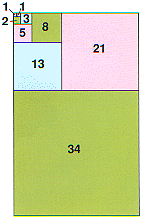Fibonacci Numbers

The first ten Fibonacci numbers are –
1, 1, 2, 3, 5, 8, 13, 21, 34, 55...

You generate Fibonacci numbers by adding together the two previous numbers in the sequence –
1 + 1 = 2
1 + 2 = 3
2 + 3 = 5
3 + 5 = 8
5 + 8 = 13
– and so on.

Fibonacci numbers are named after Leonardo Fibonacci of Pisa who lived in 13th century Italy. His description of the numbers appears in a book called Liber Abaci and makes use of an example about the rate at which rabbits breed. The numbers have so many interesting mathematical properties that they have become quite famous.

Lucas Numbers are similar to Fibonacci numbers.Squares equal in size to the Fibonacci numbers fit together to make this pattern.

Based on the book Numbers: Facts, Figures & Fiction.